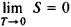# Third Law of Thermodynamics

(redirected from 3rd law of Thermodynamics)
Also found in: Dictionary, Thesaurus.

## third law of thermodynamics

[′thərd ′lȯ əv ¦thər·mō·də′nam·iks]
(thermodynamics)
The entropy of all perfect crystalline solids is zero at absolute zero temperature.
McGraw-Hill Dictionary of Scientific & Technical Terms, 6E, Copyright © 2003 by The McGraw-Hill Companies, Inc.
The following article is from The Great Soviet Encyclopedia (1979). It might be outdated or ideologically biased.

## Third Law of Thermodynamics

a law of thermodynamics according to which the entropy S of any system approaches a finite limit, which is independent of pressure, density, or phase, as the temperature T approaches absolute zero. The third law of thermodynamics, which was first stated by W. Nernst in 1906, makes it possible to determine the absolute value of entropy; such a determination is not possible in the framework of classical thermodynamics, which is based on the first and second laws of thermodynamics. In classical thermodynamics entropy can be determined only with an accuracy of an arbitrary additive constant S0. In practice, this situation does not impede most ther-modynamic studies, since the entropy difference ΔS between various states is the quantity that is actually measured. According to the third law of thermodynamics, ΔS→0 as T→0.

According to a statement of the third law advanced by M. Planck in 1911, the entropy of all bodies approaches zero as the temperature approaches absolute zero:Thus, S0 = 0, and the absolute value of entropy and other ther-modynamic potentials can be determined. Planck’s formulation corresponds to the determination of entropy in statistical mechanics in terms of the thermodynamic probability W of the state of a system: S = k In W. At a temperature of absolute zero the system is in the quantum-mechanical ground state if that state is nondegenerate. For a nondegenerate ground state W = 1; that is, the state is realized by a single microscopic distribution. Consequently, the entropy S at T = 0 is equal to zero. Actually, in all measurements, as T → 0, the entropy becomes nearly zero before the discreteness of the quantum levels of a macroscopic system becomes important. Such discreteness results in quantum degeneracy.

It follows from the third law of thermodynamics that a temperature of absolute zero cannot be attained in any finite process associated with a change in entropy and that a temperature of absolute zero can be approached only asymptotically. Therefore, the third law is sometimes formulated as the principle of nonattainability of the absolute zero of temperature. A number of thermodynamic consequences follow from the third law. For example, as T → 0, specific heats at constant pressure and constant volume, the thermal expansion coefficient, and certain similar quantities should approach zero. The validity of the third law of thermodynamics was at one time subjected to doubt, but later it was found that all apparent contradictions, such as the nonzero entropy of a number of substances at T = 0, were associated with metastable states of matter. Such states cannot be considered thermodynamic equilibrium states.

### REFERENCES

Klein, M. “Zakony termodinamiki.” In the collection Termodinamika neobratimykh protsessov: Lektsii v letnei mezhdunarodnoishkole fiziki im. E. Fermi. Moscow, 1962. (Translated from English.)

D. N. ZUBAREV